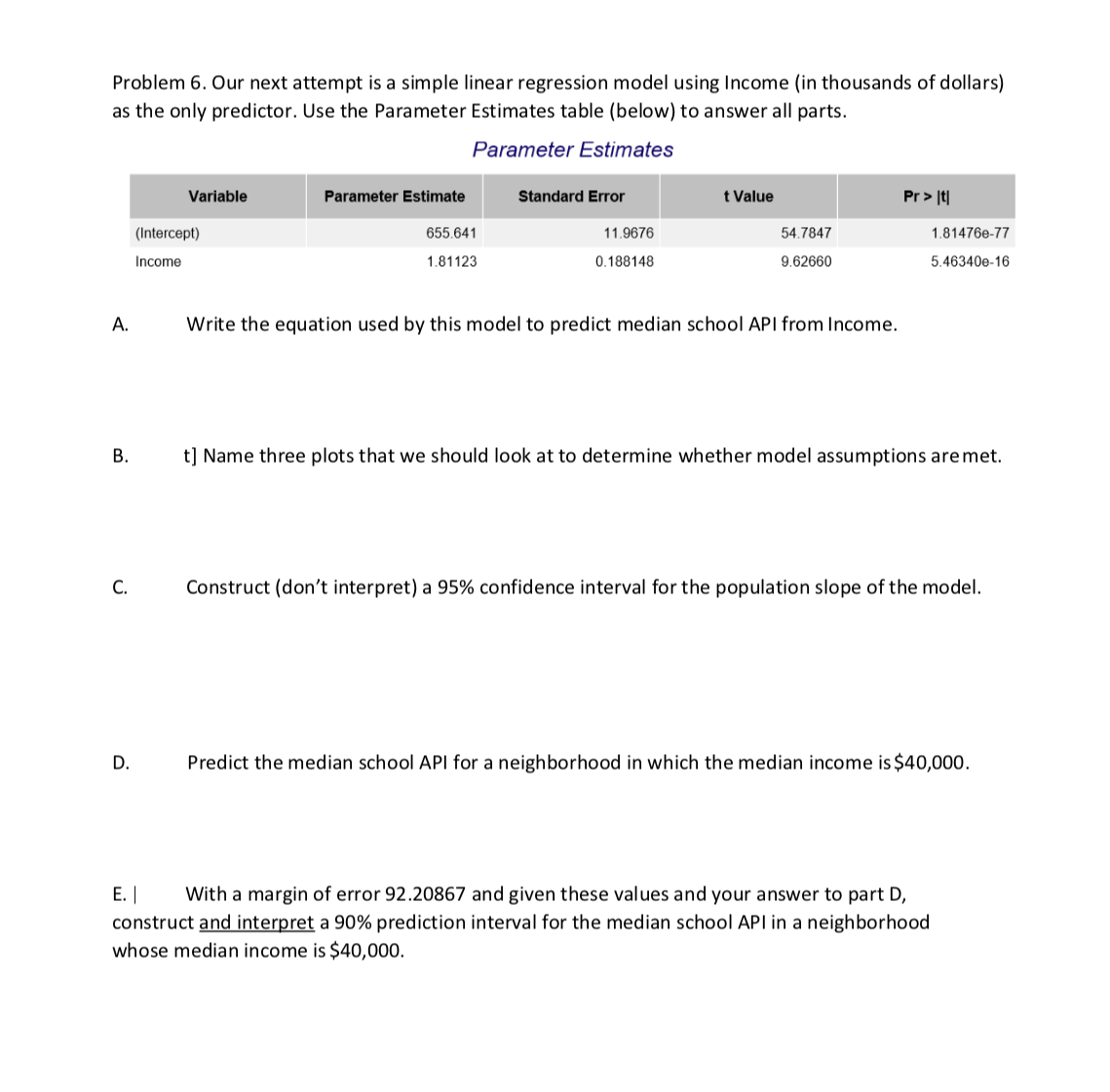# Problem 6. Our next attempt is a simple linear regression model using Income (in thousands of dollars)as the only predictor. Use the Parameter Estimates table (below) to answer all parts.Parameter EstimatesVariablet ValuePr >Parameter EstimateStandard Error655.64111.967654.7847(Intercept)1.81476e-771.811230.1881489.626605.46340e-16IncomeA.Write the equation used by this model to predict median school API from Income.t] Name three plots that we should look at to determine whether model assumptions are met.Construct (don't interpret) a 95% confidence interval for the population slope of the modelPredict the median school API for a neighborhood in which the median income is \$40,000.D.E.With a margin of error 92.20867 and given these values and your answer to part D,construct and interpret a 90% prediction interval for the median school API in a neighborhoodwhose median income is \$40,000

Question
1 viewshelp_outlineImage TranscriptioncloseProblem 6. Our next attempt is a simple linear regression model using Income (in thousands of dollars) as the only predictor. Use the Parameter Estimates table (below) to answer all parts. Parameter Estimates Variable t Value Pr > Parameter Estimate Standard Error 655.641 11.9676 54.7847 (Intercept) 1.81476e-77 1.81123 0.188148 9.62660 5.46340e-16 Income A. Write the equation used by this model to predict median school API from Income. t] Name three plots that we should look at to determine whether model assumptions are met. Construct (don't interpret) a 95% confidence interval for the population slope of the model Predict the median school API for a neighborhood in which the median income is \$40,000. D. E.With a margin of error 92.20867 and given these values and your answer to part D, construct and interpret a 90% prediction interval for the median school API in a neighborhood whose median income is \$40,000 fullscreen
check_circle

Step 1

Note:

Hey there! Thank you for posting the question. As the question you have posted has more than 3 parts, we have solved the first 3 parts for you. If you need help with any other part, please re-post the question and mention the parts you need solved.

You have not mentioned the sample size, which is necessary to find the confidence interval in part C. We have used trial-and error method to find the sample size and solved the problem using that sample size. However, if the sample size (n) we have obtained does not match with that in your original data, please replace the actual value of n in the formula we have provided, to get the answer. Henceforth, please mention the sample size in such problems.

Step 2

Part A:

Denote y as the response variable, median school API and x as the predictor, income. In the given output, the column “Parameter Estimate” gives the coefficient corresponding to the variable under the “Variable” column.

The ‘estimate’ of “Intercept” is the intercept of the regression model, which is 655.641. The coefficient of “Income” is the slope, which is given as 1.81123. Using these values, the equation for predicting y using x is: y = 655.641 + 1.81123 x.

Step 3

Part B:

Three plots that can be used to determine whether the model assumptions are met are:

• Plot of residuals versus the fitted values: The residuals (obtained by subtracting the predicted value using the equation from the observed value) are plotted against the fitted or predicted values of y. A random distribution of the plots suggests that the errors are indeed random in nature with respect to the response variable. It is not desirable to have a pattern of the residual plot.
• Plot of residuals versus the predictor: The residuals are plotted against the predictor variable values, that is, the values of x. Similar to the previous plot, a random distrib...

### Want to see the full answer?

See Solution

#### Want to see this answer and more?

Solutions are written by subject experts who are available 24/7. Questions are typically answered within 1 hour.*

See Solution
*Response times may vary by subject and question.
Tagged in

### Statistics